## Example Questions

### Example Question #1 : Sets

The product of two integers is 14. Which of the following could be the average of the two numbers?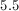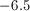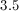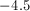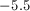Explanation:

The two integers in this case, and their respective averages, could be: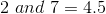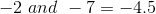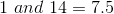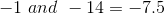Onlyis one of the answer choices.

### Example Question #1 : Sets

Which of these is a natural number?All of these are natural numbers.

None of these are natural numbers .Explanation:

The natural numbers are the positive integers (whole numbers) starting with 1.

### Example Question #2 : How To Find The Missing Number In A Set

Which of the following pairs of events are mutually exclusive?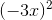for allvalues, the numbers greater thanthe positive numbers, the numbers less than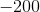the even numbers, the numbers greater thanthe numbers less than, the numbers greater thanthe negative numbers,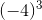the positive numbers, the numbers less thanExplanation:

We can think of mutually exclusive in terms of a Venn diagram.  We are looking for the pair of events that has nothing in common.  The only sets that don't have a single number in common are the positive numbers and the numbers less than –200.

### Example Question #1 : Sets

In the set of positive, distinct integers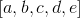, the median is. What is the minimum value of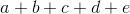?Explanation:

We know that all the numbers are positive, so they are greater than zero.  We also know that the numbers are distinct, so they are all unique.

We can write this as {a + b + 8 + d + e}, so let a and b be 1 and 2 respectively, the smallest possible positive, distinct integers. Then let d and e be 9 and 10, the smallest positive, distinct integers larger than 8.

We add the set {1 + 2 + 8 + 9 + 10} to find it equals 30.

### All GRE Math Resources